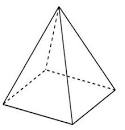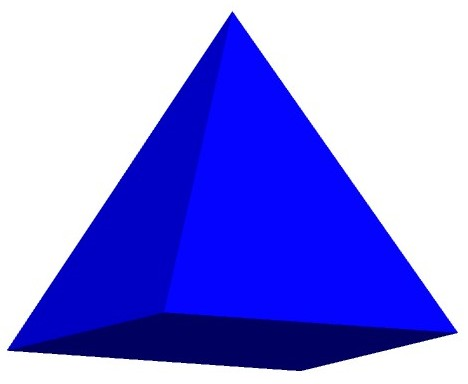How to Calculate and Solve for the Height, Base Edge and Area of a Square Pyramid | Nickzom CalculatorThe image above is a square pyramid.

To compute the area of a square pyramid, two essential parameters are needed and this parameters are base edge (a) and height (h).

The formula for calculating the area of a square pyramid:

A = a² + 2a√(( / 4) + h²)

Where;
A = Area of a square pyramid
a = Base edge of the square pyramid
h = Height of the square pyramid

Let’s solve for an example;
Find the area of a square pyramid when the base edge (a) given is 8 cm with a height (h) of 12 cm.

This implies that;
a = Base edge of the square pyramid = 8 cm
h = Height of the square pyramid = 12 cm

A = a² + 2a√(( / 4) + h²)
A = 8² + 2 x 8√(( / 4) + 12²)
A = 64 + 16√((64 / 4) + 144)
A = 64 + 16√(16 + 144)
A = 64 + (16)(√160)
A = 64 + (16)(12.65)
A = 64 + 202.3857
A = 266.386

Therefore, the area of the square pyramid is 266.386 cm2.

How to Calculate and Solve for the Height, Base Edge and Volume of a Square Pyramid | The Calculator EncyclopediaThe image above represents a square pyramid.
To compute the volume of a square pyramid requires two essential parameters which are the base edge and height of the square pyramid.

The formula for computing the volume of a square pyramid is:

V = ha² / 3

Where:
V = Volume of the Square Pyramid
a = Base edge of the Square Pyramid
h = Height of the Square Pyramid

Let’s solve an example
Find the volume of a square pyramid with a base edge of 6 cm and a height of 11 cm.

This implies that:
a = base edge of the square pyramid = 6
h= = height of the square pyramid = 11

V = ha² / 3
V = 11(6)² / 3
V = 11(36) / 3
V = 396 / 3
V = 132

Therefore, the volume of the square pyramid is 132 cm3.

Calculating the Base edge of a square pyramid when Volume and Height are Given

The formula is a = √(3V / h)

Where;
V = Volume of the Square Pyramid
a = Base edge of the Square Pyramid
h = Height of the Square Pyramid

Let’s solve an example:
Find the base edge of a square pyramid with a volume of 50 cm3 and a height of 20 cm.

This implies that;
V = Volume of the square pyramid = 50 cm3
h  = height of the square pyramid = 20 cm

a = √(3V / h)
a = √(3(50) / 20)
a = √(150 / 20)
a = √7.5
a = 2.739

Therefore, the base edge of the square pyramid is 2.739 cm.

Calculating the height of a square pyramid when Volume and Base edge are Given

The formula is h = 3V / a2

Where;
a = Base edge of the square pyramid
V = Volume of the Square Pyramid
h = Height of the Square Pyramid

Let’s solve an example:
Find the height of a square pyramid with a volume of  250 cm3  and a base edge of 7 cm2

This implies that;
V = Volume of the square pyramid = 250 cm3
a = Base edge of the square pyramid = 7 cm

h =  3V /
h = 3(250) /
h = 750 / 49
h = 15.306

Therefore, the height of the square pyramid is 15.306 cm.